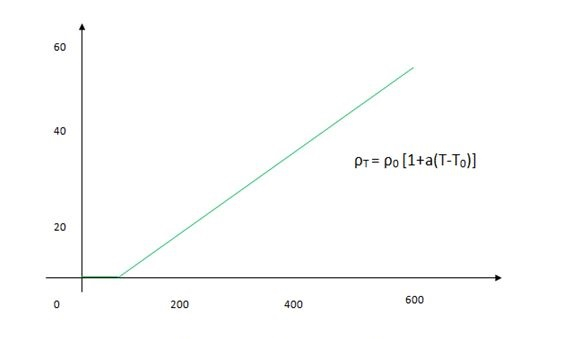# Temperature Dependence Resistance

## Introduction

Resistance is an obstacle that is present in an object with the flow of electrons. Objects with a lower amount of resistance can flow a higher amount of electrons. The significance of the current that is provoked strictly counts on the aspect of the item. Any item resists the flow of electric control which is named resistance. The dependency resistance is followed by the conductor’s geometry and it is as well as what the conductor is constructed from. However, it also relies on temperature.

## The Concept of Electrical Resistance

The application of resistance can be found with the application of different conductors that is helping the electrons in their movements. Therefore, some electrical devices like batteries and cells are managing the potential difference while the circuits are referred to as the voltage sources. The source of the supplied voltages occurs with the connection that is established across the field of connection and causes a current.

The conductor of the electric source can make the construction of the field that is creating an electric charge. The resistance is followed when the changes occur in the properties with temperature and it is varied in with the flow of the electric field (Rusdiana, 2020). In silicon material, the resistivity of the temperature coefficient is negative, representing that as temperature enlargements, the resistance goes down and it can be vice versa in some materials.## Temperature Dependence of Resistance

The temperature dependence of resistance can be comprehended by the application of the temperature model with the consideration of a simple resistance model. In order to comprehend the knowledge-gaining process is making ensure that the resistivity of temperature is varied from the linear on the temperature conductor. Particles and molecules restrain electrons streaming via a conductor (Shaaban et al. 2020). With the understanding gained from the unit of resistance, it can be declared that the area and the length do not transform as temperature shifts.

Images Coming soon

With the comprehension of the process of how the resistivity works, the linear temperature influences to make a variation in the transformation of the temperature. In physics, the character R represents the resistance, and the terms are written with the equation of $\mathrm{R=R_{0}(1+\alpha \Delta T)}$. This equation, $\mathrm{\alpha}$ represents the resistivity of the coefficient temperature.

## Unit of Resistance

A resistor with an opposition that is almost autonomous of temperature creates exploitation. The unit of resistance means it is allowing one current unit to flow through the potential unit distinction. The unit of the resistance is called ohm per volt.

### Conductors

The materials offer very low resistance to the lower rate of flowing electrons. Aluminium is one of the most conductors that is known as good conductor of the lower cost of abundance.

### Insulators

Those items offer a very high amount of resistance to the electron's flow. These items are very conductors of inadequat electricity and are primarily used in electric techniques to control the current leakage.

## Resistivity vs Temperature

Images Coming soon

According to the facts of physics, it has been observed that the resistivity of a component generally relies on the temperature. This dependency can be represented by a mathematical expression such as $\mathrm{\rho t=\rho _{0}[1+\alpha (T-T_{0})]}$. this particular mathematical representation of the formula help to represent the relationship lies between temperature and resistivity. In this formula, $\mathrm{\rho{0}}$ represent the resistivity at a certain temperature that is considered a standard one. $\mathrm{P_{t}}$ is considered as the representative of resistivity at $\mathrm{t^{0}}$ C and $\mathrm{T_{0}}$ stands for the reference temperature. $\mathrm{\alpha}$ represents the temperature coefficient of the resistivity.

## Resistance vs Temperature

In the case of normal conductors, the conduction and the valence generally overlap each other. For this reason, the conduction band of a conductor has excess electrons. The more energy will be observed, the more electrons will go to the conduction band from the valence band when the temperature is raised. In addition, it has been seen that in the case of semiconductors, the more the temperature will increase; the more material will increase accordingly. The outermost electrons in accordance with the increment level of the temperature will acquire the energy as well. The acquirement of the energy results in leaving the outermost electrons.

## Conclusion

The present tutorial has cast light on the discussion that focuses on the definition of electrical resistance. Furthermore, the tutorial has included a section where the relation between temperature and resistance has been stated. It has been seen that the temperature is directly proportional to the resistance. It explains that when in conductors, the resistance will be increased, an increment in temperature is identified and the reduction of resistance decreases the temperature as well. Along with this, the tutorial further explains the factors regarding the relation of resistivity and resistance with temperature.

## FAQs

Q.1. What is resistance?

Ans. The word resistance can be defined as the measurement of a current flow that is opposite of the direction of the normal flow of current in a circuit of electricity. The resistance is represented by the Greek letter omega which is symbolically represented by $\mathrm{\Omega }$.

Q.2. What is the relation between resistance and temperature coefficient?

Ans. The resistance-change factor based on per degree celsius of temperature is generally termed as temperature coefficient of resistance. This specific factor is represented by the symbol of $\mathrm{\alpha}$, named alpha.

Q.3. Why does the temperature increase with the increment of resistance?

Ans. The core reason behind the increment in temperature in accordance with the increment of resistance is that the thermal velocity of a free-electron orbiting a nucleus increases helps to increase the temperature of that certain place as well.

Q.4. What is temperature-dependent?

Ans. The dependence of temperature is represented by the symbol of $\mathrm{\mu}$ that depends on two factors, such as scattering of the photon and ionized impurity scattering.